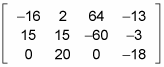##### Linear Algebra For Dummies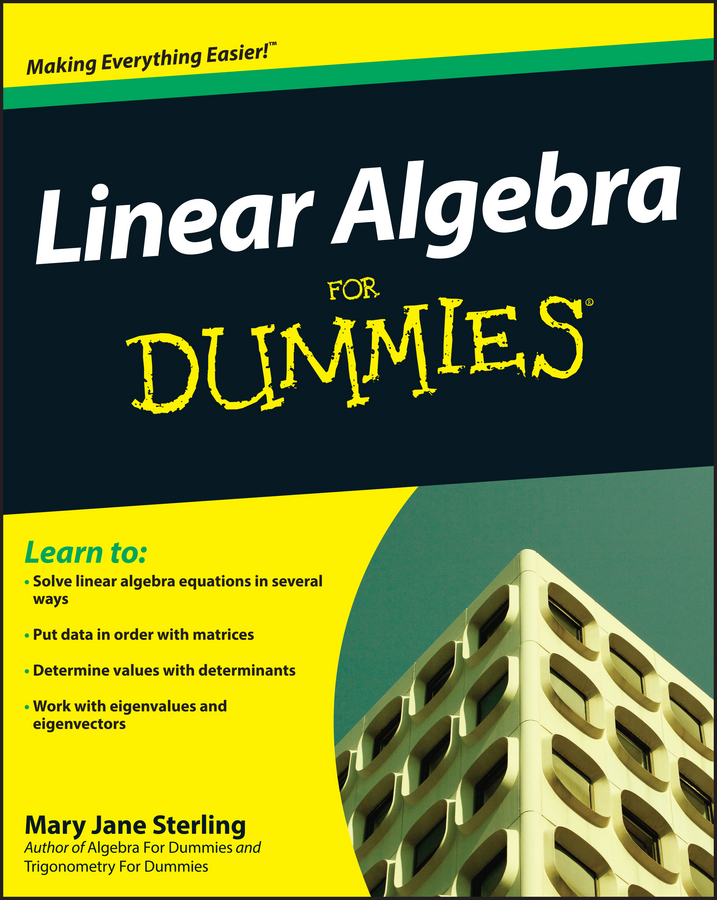Multiplying matrices is very useful when solving systems of equations. This is because you can multiply a matrix by its inverse on both sides of the equal sign to eventually get the variable matrix on one side and the solution to the system on the other.

Unfortunately, multiplying two matrices together is not as simple as multiplying the corresponding terms. Each element of each matrix gets multiplied by each term of the other at some point.

For matrix multiplication, the matrices are written right next to each other with no symbol in between. If you want to multiply matrices A and B to get their product AB, the number of columns in A must match the number of rows in B. Each element in the first row of A is multiplied by each corresponding element from the first column of B, and then all of these products are added together to give you the element in the first row, first column of AB. This is known as taking the dot product of the first row of A with the first column of B. To find the value in the first row, second column position, take the dot product of the first row of A with the second column of B by multiplying each element in the first row of A by each element in the second column of B, and then add all of these products together. In the end, after all the possible dot products are computed, your new matrix should have the same number of rows as A and the same number of columns as B.

For example, to multiply a matrix A with 3 rows and 2 columns by a matrix B with 2 rows and 4 columns, you take the dot product of the first row of A with each of the columns of B, producing 4 terms in the first row of the product AB. Taking the dot product of the second row of A with each of the columns of B produces the second row of the product AB, which contains another 4 terms. And the same goes for producing the last row of AB. You end up with a matrix of 3 rows and 4 columns.

If matrix A has dimensions m x n and matrix B has dimensions n x p, AB is an m-x-p matrix. This figure gives you a visual representation of matrix multiplication.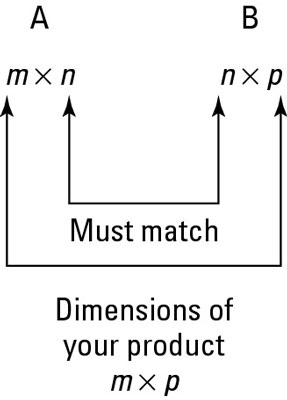Multiplying two matrices that match up.

When you multiply matrices, you don't multiply the corresponding parts like when you add or subtract. Also, in matrix multiplication, AB doesn't equal BA. In fact, just because you can multiply A by B doesn't mean you can multiply B by A. The number of columns in A may be equal to the number of rows in B, but the number of columns in B may not equal the number of rows in A. For example, you can multiply a matrix with 3 rows and 2 columns by a matrix with 2 rows and 4 columns. However, you can't do the multiplication the other way because you can't multiply a matrix with 2 rows and 4 columns by a matrix with 3 rows and 2 columns. If you tried taking the dot product by multiplying the correct terms together and then adding their products, somewhere along the way you would run out of terms!

Here's an example of matrix multiplication. Say a problem asks you to multiply the following two matrices: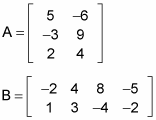First, check to make sure that you can multiply the two matrices. Matrix A is 3 x 2 and B is 2 x 4, so you can multiply them to get a 3-x-4 matrix as an answer. Now you can proceed to take the dot product of every row of the first matrix with every column of the second.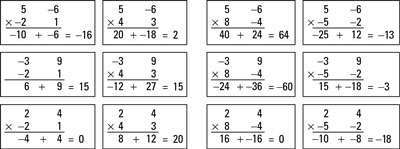The process of multiplying AB.

This figure lays out the process for you. You can start by multiplying each term in the first row of A by the sequential terms in the columns of matrix B. Note that multiplying each entry in row one by the corresponding entry in column one and adding these products together gives you row one, column one's entry. Similarly, multiplying each entry in row two by the corresponding entry in column three gives you row two, column three's entry.

Taking out all the fluff, the product matrix is